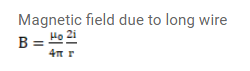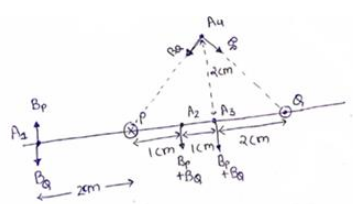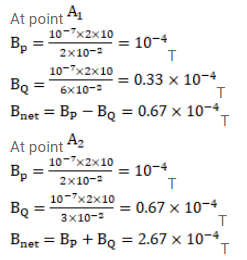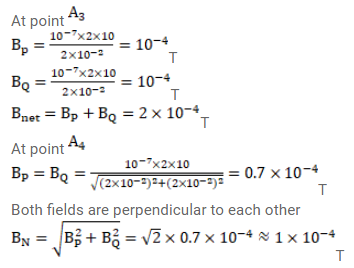# Figure shows two parallel wires separated by a distance of

Question:

Figure shows two parallel wires separated by a distance of $4.0 \mathrm{~cm}$ and carrying equal currents of $10 \mathrm{~A}$ along opposite directions. Find the magnitude of the magnetic field $B$ at points $A_{1}, A_{2}, A_{3}$ and $A_{4}$.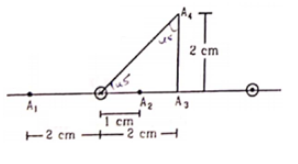Solution: